cancel
Showing results for
Did you mean:Helper I

## How to get the difference between more rows?

Hey guys,

i need your help please i hava a huge table with lot of data (many excel files in one folder) and i want the differences between the Sources (excelfiles).

I have found something which is almost good for that what i want in the Quick Measures:

Value difference from LIST_211022_MOT_PROG_P2022-01_final.xlsx =
VAR __BASELINE_VALUE =
CALCULATE(
SUM('Zusammenfassung'[Value]),
'Zusammenfassung'[Source.Name]
IN { "LIST_211022_MOT_PROG_P2022-01_final.xlsx" }
)
VAR __MEASURE_VALUE = SUM('Zusammenfassung'[Value])
RETURN
IF(NOT ISBLANK(__MEASURE_VALUE), __MEASURE_VALUE - __BASELINE_VALUE)

But the problem here ist that the Source is not dinamic, i have to filter between the differen excel files and need that den differences.

Almost like the function in the pivot tabel (show values as difference).

2 ACCEPTED SOLUTIONSSuper User

@KimBoehmer
If you want to follow my suggestion and disply the results in a tablevisual, then place the Sorce Name column in the table along with the following measure. From the visual format options, activate the total and rename it "Difference"

``````Value =
VAR MeasureValue =
SUM ( 'Zusammenfassung'[Value] )
VAR T1 =
VALUES ( 'Zusammenfassung'[Source.Name] ),
"@Value", CALCULATE ( SUM ( 'Zusammenfassung'[Value] ) )
)
VAR DifferenceValue =
MAXX ( T1, [@Value] ) - MINX ( T1, [@Value] )
RETURN
IF (
HASONEVALUE ( 'Zusammenfassung'[Source.Name] ),
MeasureValue,
DifferenceValue
)``````

If you wish to display the difference in a seperate chart, then place the following measure in the chart ALONE (don't place any column along with it in the same chart)

``````Value difference =
VAR T1 =
VALUES ( 'Zusammenfassung'[Source.Name] ),
"@Value", CALCULATE ( SUM ( 'Zusammenfassung'[Value] ) )
)
RETURN
MAXX ( T1, [@Value] ) - MINX ( T1, [@Value] )``````Super User

Hi @KimBoehmer
Please refer to attached sample file with the solution. I've created a new column using power query to get the Source Number for sorting purposes.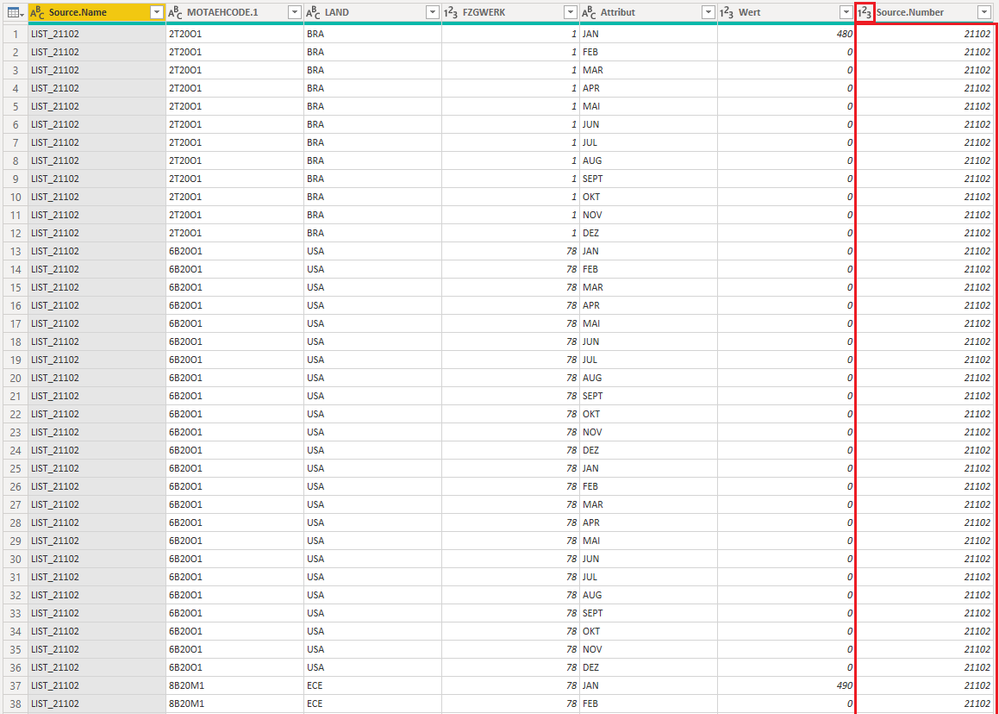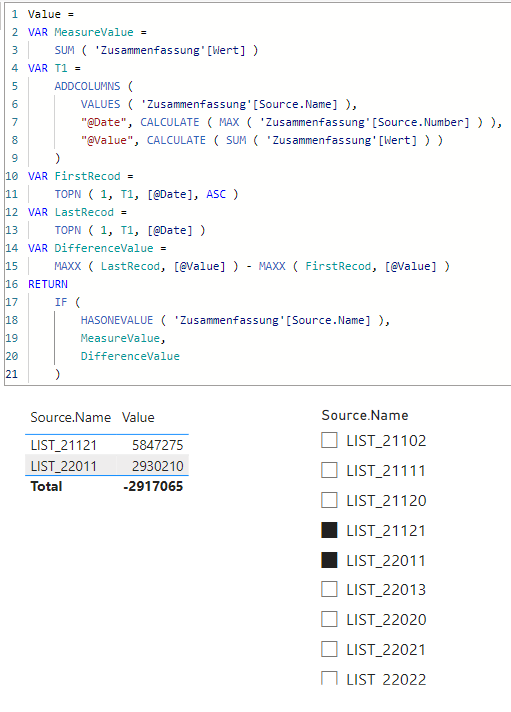``````Value =
VAR MeasureValue =
SUM ( 'Zusammenfassung'[Wert] )
VAR T1 =
VALUES ( 'Zusammenfassung'[Source.Name] ),
"@Date", CALCULATE ( MAX ( 'Zusammenfassung'[Source.Number] ) ),
"@Value", CALCULATE ( SUM ( 'Zusammenfassung'[Wert] ) )
)
VAR FirstRecod =
TOPN ( 1, T1, [@Date], ASC )
VAR LastRecod =
TOPN ( 1, T1, [@Date] )
VAR DifferenceValue =
MAXX ( LastRecod, [@Value] ) - MAXX ( FirstRecod, [@Value] )
RETURN
IF (
HASONEVALUE ( 'Zusammenfassung'[Source.Name] ),
MeasureValue,
DifferenceValue
)``````
22 REPLIES 22Super User

Hi @KimBoehmer
Please refer to attached sample file with the solution. I've created a new column using power query to get the Source Number for sorting purposes.``````Value =
VAR MeasureValue =
SUM ( 'Zusammenfassung'[Wert] )
VAR T1 =
VALUES ( 'Zusammenfassung'[Source.Name] ),
"@Date", CALCULATE ( MAX ( 'Zusammenfassung'[Source.Number] ) ),
"@Value", CALCULATE ( SUM ( 'Zusammenfassung'[Wert] ) )
)
VAR FirstRecod =
TOPN ( 1, T1, [@Date], ASC )
VAR LastRecod =
TOPN ( 1, T1, [@Date] )
VAR DifferenceValue =
MAXX ( LastRecod, [@Value] ) - MAXX ( FirstRecod, [@Value] )
RETURN
IF (
HASONEVALUE ( 'Zusammenfassung'[Source.Name] ),
MeasureValue,
DifferenceValue
)``````Helper I

Great thank you ver much 🙂Super User

Hi @KimBoehmer

``````Value difference =
VAR __MEASURE_VALUE =
SUM ( 'Zusammenfassung'[Value] )
RETURN
SUMX (
VALUES ( 'Zusammenfassung'[Source.Name] ),
VAR __BASELINE_VALUE =
CALCULATE ( SUM ( 'Zusammenfassung'[Value] ) )
RETURN
IF ( NOT ISBLANK ( __MEASURE_VALUE ), __MEASURE_VALUE - __BASELINE_VALUE )
)``````Helper I

Hi,

Unfortunately this ist working.Super User

How does your visual look like? What results did you get?Helper I

Hi,

i get no result, there is no mistake in the formular.

and i have a slicer with the different Sources, i only want to compare two different sources at the same time.Super User

So you want to select two sources from the slicer and the display the values of the two sources in addition to the difference between them? Is that correct? If so, I would suggest to use a table visual as it has a total where we csn disply the difference value.Helper I

Here the overview with your formular in comparison:Super User

@KimBoehmer
If you want to follow my suggestion and disply the results in a tablevisual, then place the Sorce Name column in the table along with the following measure. From the visual format options, activate the total and rename it "Difference"

``````Value =
VAR MeasureValue =
SUM ( 'Zusammenfassung'[Value] )
VAR T1 =
VALUES ( 'Zusammenfassung'[Source.Name] ),
"@Value", CALCULATE ( SUM ( 'Zusammenfassung'[Value] ) )
)
VAR DifferenceValue =
MAXX ( T1, [@Value] ) - MINX ( T1, [@Value] )
RETURN
IF (
HASONEVALUE ( 'Zusammenfassung'[Source.Name] ),
MeasureValue,
DifferenceValue
)``````

If you wish to display the difference in a seperate chart, then place the following measure in the chart ALONE (don't place any column along with it in the same chart)

``````Value difference =
VAR T1 =
VALUES ( 'Zusammenfassung'[Source.Name] ),
"@Value", CALCULATE ( SUM ( 'Zusammenfassung'[Value] ) )
)
RETURN
MAXX ( T1, [@Value] ) - MINX ( T1, [@Value] )``````Helper I

Thank you very much, but i have one last question 🙂

The difference is always postiv but i have positiv an negativ chnages in the numbers how can i display them in a chart?Super User

@KimBoehmer
Yes I made it this way as there is no logic (at least from my prespective) that defines which one minus which one. Please clarify this point.Helper I

i want to compare the volumes from 2021 to 2022 for each month and of course some month are better than last year and others not. Therefore i would need plus and minus changesSuper User

Is there a date or year-month column that defines the date of each list?

Do you to subtract the latest minus the earliest or the opposite?Helper I

Yes i have the month and yes i will substract the latest minus the erliest i have filtered.Helper ISuper User

Apologies for the late response. I was extremely busy tody I didn't have the chance to look into it.
Actually, this shouldn't be a problem we just need to filter the table or sort it by date then retrieve the amount for both max and min dates.

``````Value =
VAR MeasureValue =
SUM ( 'Zusammenfassung'[Value] )
VAR T1 =
VALUES ( 'Zusammenfassung'[Source.Name] ),
"@Date", CALCULATE ( MAX ( 'Zusammenfassung'[Date] ) ),
"@Value", CALCULATE ( SUM ( 'Zusammenfassung'[Value] ) )
)
VAR FirstRecod =
TOPN ( 1, T1, [@Date], ASC )
VAR LastRecod =
TOPN ( 1, T1, [@Date] )
VAR DifferenceValue =
MAXX ( LastRecod, [@Value] ) - MAXX ( FirstRecod, [@Value] )
RETURN
IF (
HASONEVALUE ( 'Zusammenfassung'[Source.Name] ),
MeasureValue,
DifferenceValue
)``````

*UPDATE

The measure has been updated fixing one error @KimBoehmerHelper I

Hi @tamerj1 dont worry 🙂

i have tested your formular, but the result its unfortunately not correct 😞

Here two examples: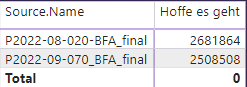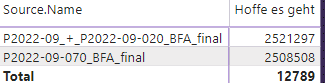BR KimSuper User

Please copy/paste the formula that you've used. Did you check the updated formula?Helper I

Yes, now it shows this: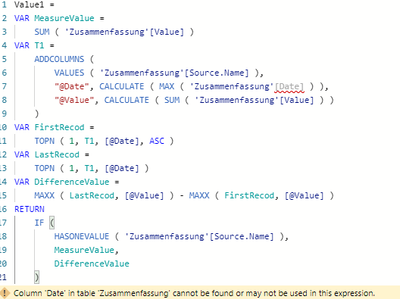Super User

For [Date] you need to use your month column. The month column has to be of YYYYMM format.